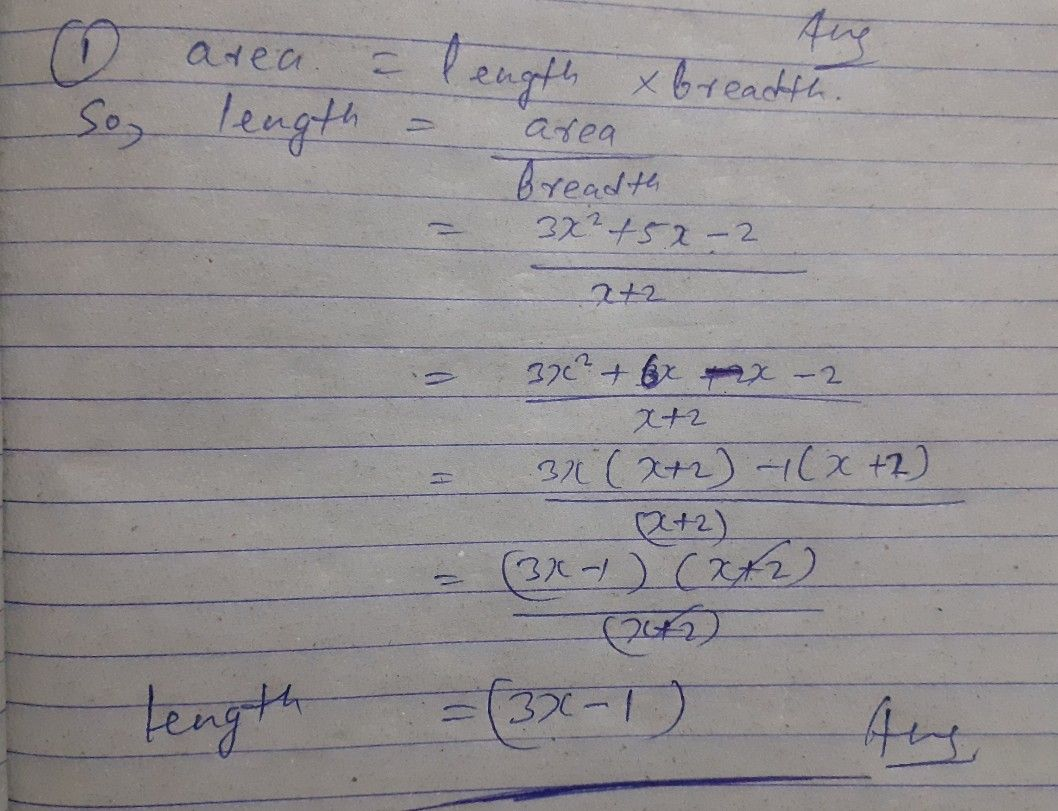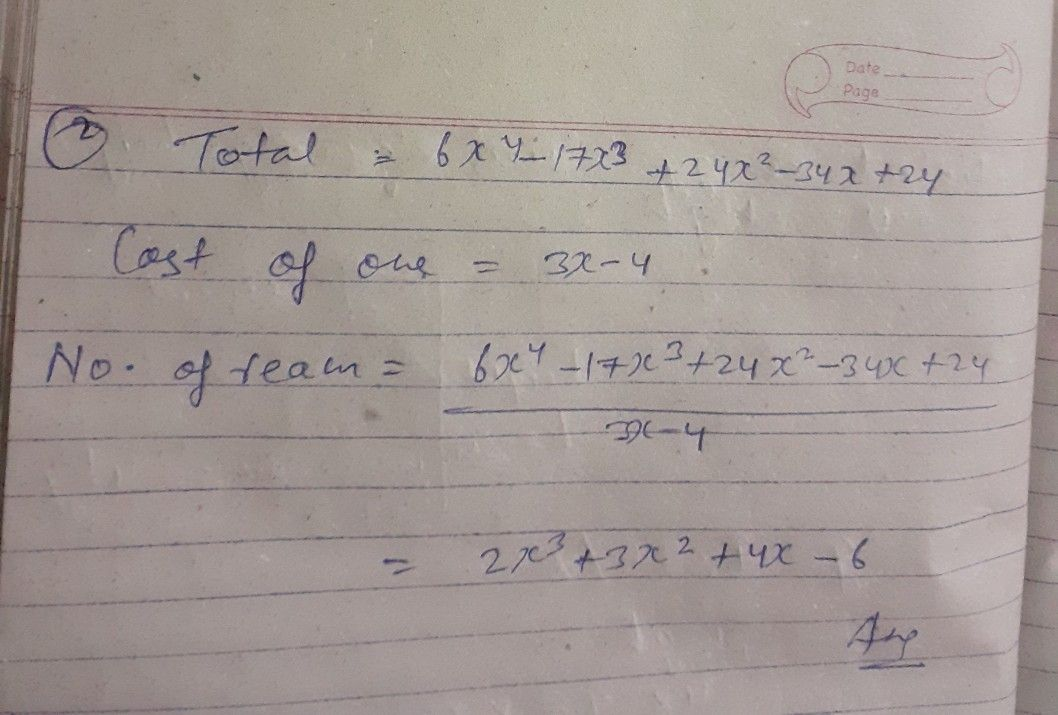Symbol
Problem$B$ Solve the following problems using factoring polynomials. $1.$ A rectangular garden in a backyard has an area $\bar{of\left(3x^{2+5x-2}\right)}$ square meters. Its width is $s\left(x+2\right)$ meters. Find the length of the garden. $2$ If one ream of bond paper $cost5\left(3x-4\right)$ $oesos$ how many reams can you buy for $\left(6x^{4}-17x^{3+24x^{2}-34x+24}\right)$ $oosos2$
10th-13th grade
Other
Search count: 127
SolutionQanda teacher - Awaneesh07